# Unexpected square wave

Last night a friend from Vanderbilt, Father John Rickert, sent me a curious math problem. (John was a PhD student in the math department while I was a postdoc. He went on to be a Catholic priest after graduating.) He said that if you look at iterates of

f(x) = exp( sin(x) )

the plots become square.

Here’s a plot to start the discussion, looking at f(x), f(f(x)), f(f(f(x))), and f(f(f(f(x)))).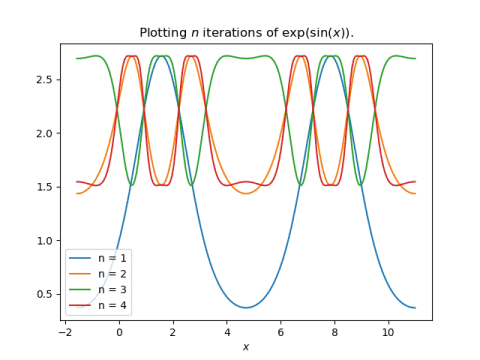One thing that is apparent is that there’s a big difference between applying f once and applying it at least two times. The former can range between 1/e and e, but the latter must be between exp(1/e) and e.

The plots overlap in a way that’s hard to understand, so let’s spread them out, plotting one iteration at a time.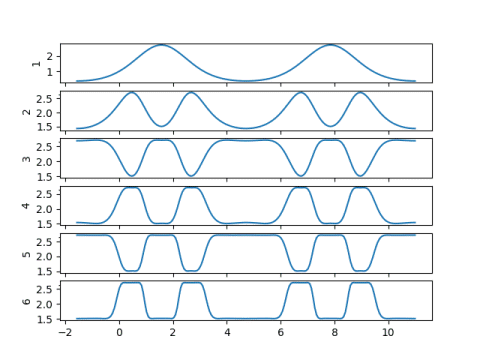Now we can see the plots becoming flatter as the number of iterations increases. We can also see that even and odd iterations are roughly mirror images of each other. This suggests we should make a plot of at just even or just odd iterates. Here we go: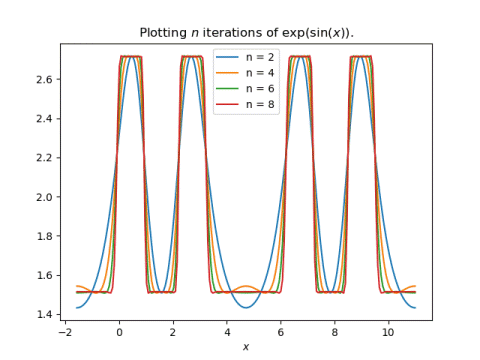This shows more clearly how the plots are squaring off pretty quickly as the number of iterations increases.

My first thought when John showed me his plot was that it must have something to do with the contraction mapping theorem. And I suppose it does, but not in a simple way. The function f(x) is not a contraction mapping for any x. But f(f(x)) is a contraction mapping for some x, and further iterates are contractions for more values of x.

Update: See the next post calculates the fixed points of f(f(x)) and demonstrates how the stable fixed points converge and the unstable fixed point does not.

My second thought was that it would be interesting to look at the Fourier transform of these iterates. For any finite number of iterations, the result is a periodic, analytic function, and so eventually the Fourier coefficients must go to zero rapidly. But I suspect they initially go to zero slowly, like those of a square wave would. I intend to update this post after I’ve had a chance to look at the Fourier coefficients.

Update: As expected, the Fourier coefficients decay slowly. I plotted the Fourier sine coefficients for f(f(f(f(x)))) using Mathematica.

    f[x_] := Exp[Sin[x]]
g[x_] := f[f[f[f[x]]]]
ListPlot[ Table[
NFourierSinCoefficient[g[x], x, i],
{i, 30}]
]


This produced the plot below.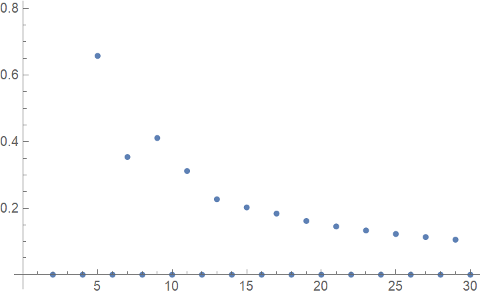The even-numbered coefficients are zero, but the odd-numbered coefficients are going to zero very slowly. Since the function g(x) is infinitely differentiable, for any k you can go far enough out in the Fourier series that the coefficients go to zero faster than nk. But initially the Fourier coefficients decay like 1/n, which is typical of a discontinuous function, like a square wave, rather than an infinitely differentiable function.

By the way, if you replace sine with cosine, you get similar plots, but with half as many flat spots per period.

# A brief comment on hysteresis

You might hear hysteresis described as a phenomena where the solution to a differential equation depends on its history. But that doesn’t make sense: the solution to a differential equation always depends on its history. The solution at any point in time depends (only) on its immediately preceding state. You can take the state at any particular time, say position and velocity, as the initial condition for the solution going forward.

But sometimes there’s something about the solution to a differential equation that does not depend on its history. For a driven linear oscillator, the frequency of the solution depends only on the frequency of the driving function. But this is not necessarily true of a nonlinear oscillator. The same driving frequency might correspond to two different solution frequencies, depending on whether the frequency of the driving function increased to its final value or decreased to that value.

Hysteresis is interesting, but it’s more remarkable that linear system does not exhibit hysteresis. Why should the solution frequency depend only on the driving frequency and not on the state of the system?

The future of system with hysteresis still depends only on its immediately preceding state. But there may be a thin set of states that lead to solutions with a given frequency, and the easiest way to arrive in such a set may be to sneak up on it slowly.

# Behold! The Brusselator!

Having watched a few episodes of Phineas and Ferb, when I see “Brusselator” I imagine Dr. Doofenschmertz saying “Behold! The Brusselator!”

But the Brusselator is considerably older than Phineas and Ferb. It goes back to Belgian scientists René Lefever and Grégoire Nicolis in 1971  who combined “Brussels” and “oscillator” to name the system after their institution, Université Libre de Bruxelles. It’s a dynamical system that has its origins in modeling chemical reactions.The phase plot below shows that as you start from multiple initial conditions, you always end up on the same limit cycle.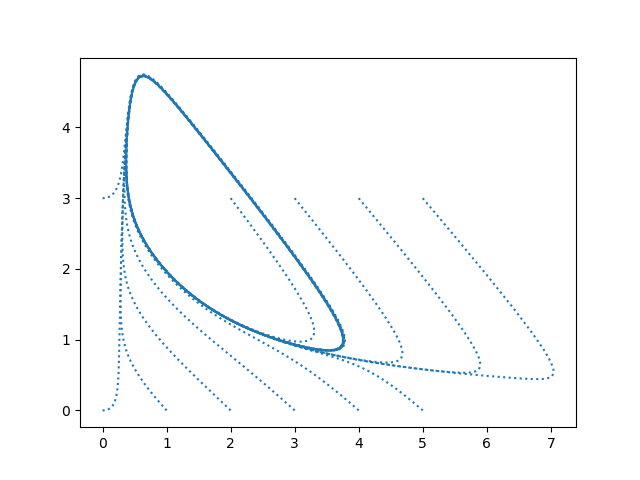Here’s the Python code that produced the plot.

    from scipy import linspace
from scipy.integrate import solve_ivp
import matplotlib.pyplot as plt

A, B = 1, 3

def brusselator(t, z):
x, y = z
return [A + x*x*y - (B+1)*x, B*x - x*x*y]

a, b = 0, 10
t = linspace(a, b, 1000)

for x0 in range(0, 6):
for y0 in [0, 3]:
sol = solve_ivp(brusselator, [a, b], [x0, y0], t_eval=t)
plt.plot(sol.y, sol.y, ":", color="tab:blue")
plt.show()


## More dynamical systems posts

 R. Lefever and G. Nicholis. Chemical instabilities and sustained oscillations. Journal of Theoretical Biology. Volume 30, Issue 2, February 1971, Pages 267-284

# A different view of the Lorenz system

The Lorenz system is a canonical example of chaos. Small changes in initial conditions eventually lead to huge changes in the solutions.

And yet discussions of the Lorenz system don’t simply show this. Instead, they show trajectories of the system, which make beautiful images, but do not demonstrate the effect of small changes to initial conditions. Or they demonstrate it in two or three dimensions where it’s harder to see.

If you’ve seen the Lorenz system before, this is probably the image that comes to mind.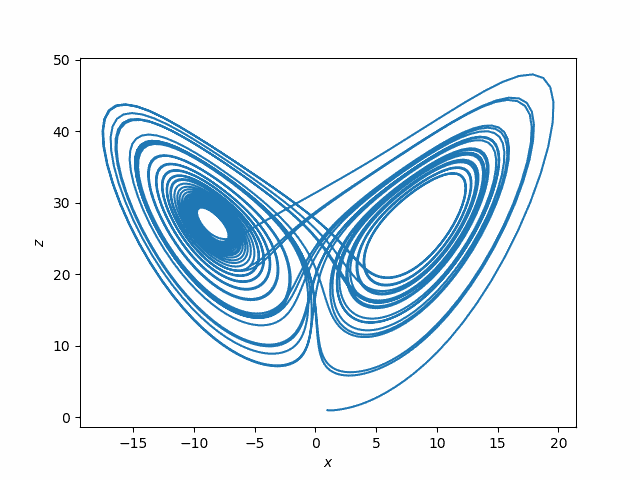This plots (x(t), z(t)) for the solutions to the system of differential equations

x‘= σ(yx)
y‘ = x(ρ – z) – y
z‘ = xy – βz

where σ = 10, ρ = 28, β = 8/3. You could use other values of these parameters, but these were the values originally used by Edward Lorenz in 1963.

The following plots, while not nearly as attractive, are more informative regarding sensitive dependence on initial conditions.In this plot, x1 is the x-component of the solution to the Lorenz system with initial condition

(1, 1, 1)

and x2 the x-component corresponding to initial condition

(1, 1, 1.00001).

The top plot is x1 and the bottom plot is x1x2.

Notice first how erratic the x component is. That might not be apparent from looking at plots such as the plot of (x, z) above.

Next, notice that for two solutions that start off slightly different in the z component, the solutions are nearly identical at first: the difference between the two solutions is zero as far as the eye can tell. But soon the difference between the two solutions has about the same magnitude as the solutions themselves.

Below is the Python code used to make the two plots.

    from scipy import linspace
from scipy.integrate import solve_ivp
import matplotlib.pyplot as plt

def lorenz(t, xyz):
x, y, z = xyz
s, r, b = 10, 28, 8/3. # parameters Lorentz used
return [s*(y-x), x*(r-z) - y, x*y - b*z]

a, b = 0, 40
t = linspace(a, b, 4000)

sol1 = solve_ivp(lorenz, [a, b], [1,1,1], t_eval=t)
sol2 = solve_ivp(lorenz, [a, b], [1,1,1.00001], t_eval=t)

plt.plot(sol1.y, sol1.y)
plt.xlabel("$x$")
plt.ylabel("$z$")
plt.savefig("lorenz_xz.png")
plt.close()

plt.subplot(211)
plt.plot(sol1.t, sol1.y)
plt.xlabel("$t$")
plt.ylabel("$x_1(t)$")
plt.subplot(212)
plt.plot(sol1.t, sol1.y - sol2.y)
plt.ylabel("$x_1(t) - x_2(t)$")
plt.xlabel("$t$")
plt.savefig("lorenz_x.png")


One important thing to note about the Lorenz system is that it was not contrived to show chaos. Meteorologist and mathematician Edward Lorenz was lead to the system of differential equations that bears his name in the course of his work modeling weather. Lorenz understandably assumed that small changes in initial conditions would lead to small changes in the solutions until numerical solutions convinced him otherwise. Chaos was a shocking discovery, not his goal.

# Cobweb plots

Cobweb plots are a way of visualizing iterations of a function.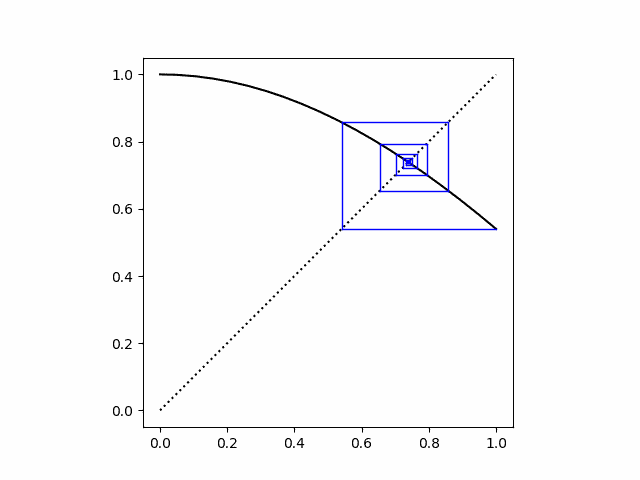For a function f and a starting point x, you plot (x, f(x)) as usual. Then since f(x) will be the next value of x, you convert it to an x by drawing a horizontal line from (x, f(x)) to (f(x), f(x)). In other words, you convert the previous y value to an x value by moving to where a horizontal line intersects the line y = x. Then you go up from the new x to f applied to the new x. The Python code below makes this all explicit.

Update: I made a couple changes after this post was first published. I added the dotted line y = x to the plots, and I changed the aspect ratio from the default to 1 to make the horizontal and vertical scales the same.

    import matplotlib.pyplot as plt
from scipy import cos, linspace

def cobweb(f, x0, N, a=0, b=1):
# plot the function being iterated
t = linspace(a, b, N)
plt.plot(t, f(t), 'k')

# plot the dotted line y = x
plt.plot(t, t, "k:")

# plot the iterates
x, y = x0, f(x0)
for _ in range(N):
fy = f(y)
plt.plot([x, y], [y,  y], 'b', linewidth=1)
plt.plot([y, y], [y, fy], 'b', linewidth=1)
x, y = y, fy

plt.axes().set_aspect(1)
plt.show()
plt.close()


The plot above was made by calling

    cobweb(cos, 1, 20)

to produce the cobweb plot for 20 iterations of cosine starting with x = 1. There’s one fixed point, and the cobweb plot spirals into that fixed point.

Next let’s look at several iterations of the logistic map f(x) = rx(1 – x) for differing values of r.

    # one fixed point
cobweb(lambda x: 2.9*x*(1-x), 0.1, 100)

# converging to two-point attractor
cobweb(lambda x: 3.1*x*(1-x), 0.1, 100)

# starting exactly on the attractor
cobweb(lambda x: 3.1*x*(1-x), 0.558, 100)

# in the chaotic region.
cobweb(lambda x: 4.0*x*(1-x), 0.1, 100)


The logistic map also has one stable fixed point if r ≤ 3. In the plot below, r = 2.9.Next we set r = 3.1. We start at x = 0.1 and converge to the two attractor points.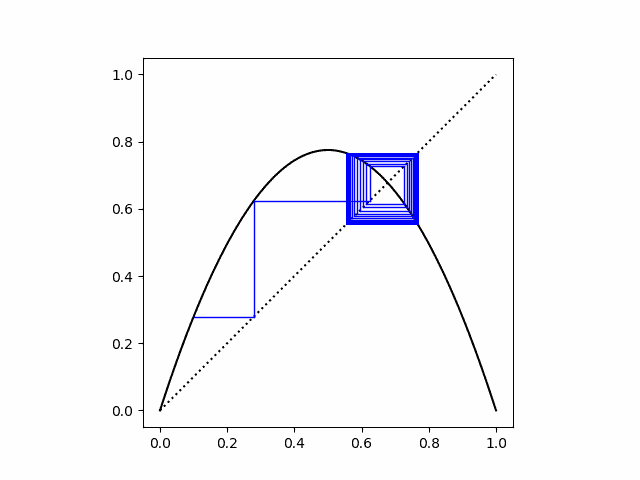If we start exactly on one of the attractor points the cobweb plot is simply a square.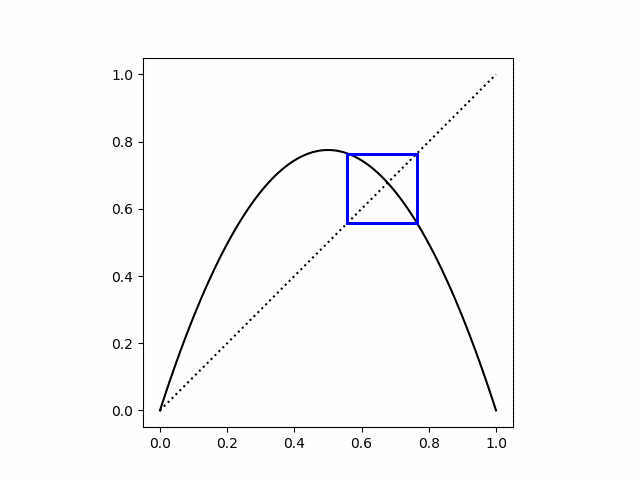Finally, when we set r = 4 we’re in the chaotic region.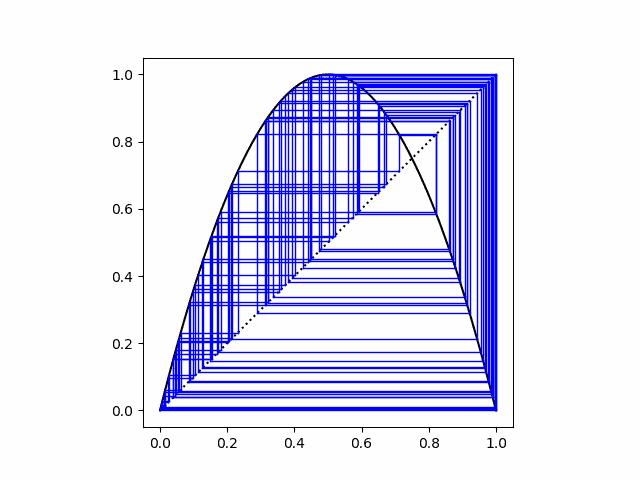# Logistic trajectories

This post is a follow-on to the post on how to make the logistic bifurcation diagram below.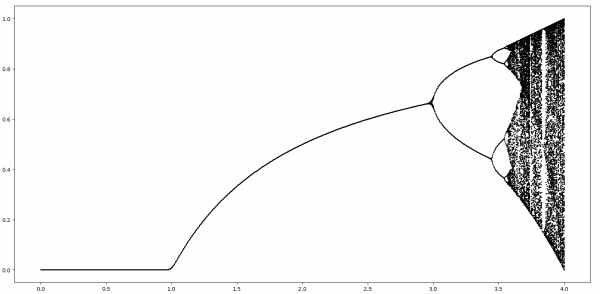That post plotting the attractors for iterations of

f(x) = r x(1 – x).

This post will plot a few trajectories over time for differing starting points and varying values of r.

For values of r less than 3, the iterations converge to the same point regardless of where they start. The fixed point only depends on r. In the plot below, r = 2.9. We start from two different initial points, x = 0.55 and x = 0.71. Both trajectories oscillate toward a common limiting value. (As explained in the earlier post, the limiting value is (r-1)/r = 0.6786.)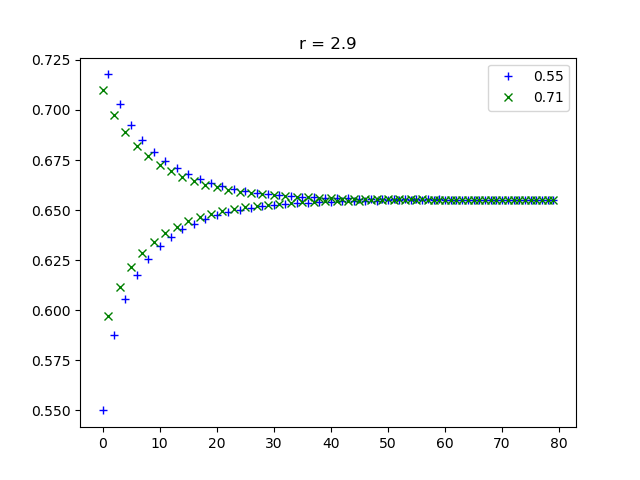For values of r a little greater than 3, the iterations oscillate between two values. Here we chose r = 3.1, and again we start from x = 0.55 and x = 0.71.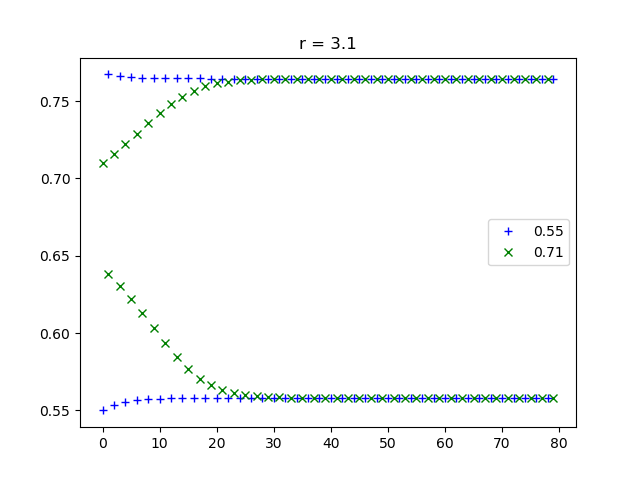For some larger value of r the iterations rotate between four values, then eight, etc. But for even larger values of r the trajectories are chaotic. Nearby starting points lead to divergent iterations. In the plot below, r = 3.7, which is in the chaotic region. We start with points close to each other, x = 0.5001 and x = 0.5002.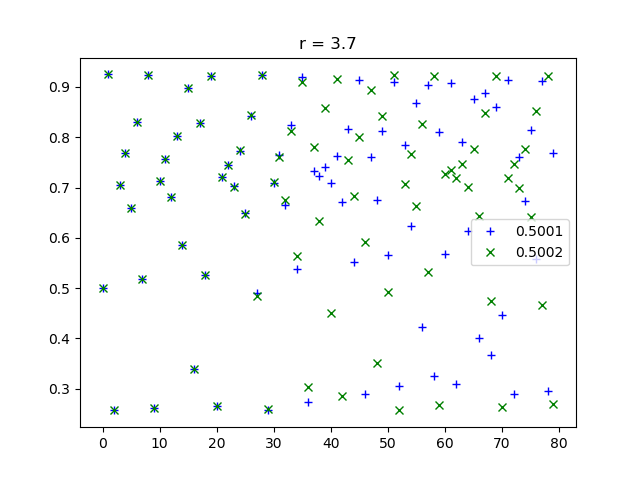Notice how the blue crosses and green x’s are on top of each other for the first 30 iterations or so, then they diverge.

These plots were created using the following Python code.

import numpy as np
import matplotlib.pyplot as plt

def f(x, r):
return r*x*(1-x)

def time_plot(x0, y0, r, name):
N = 80
x = np.zeros(N)
y = np.zeros(N)
x = x0
y = y0
for i in range(1,N):
x[i] = f(x[i-1], r)
y[i] = f(y[i-1], r)

t = np.arange(N)
plt.plot(t, x, "b+")
plt.plot(t, y, "gx")
plt.legend([str(x0), str(y0)])
plt.title(f"r = {r}")
plt.savefig(name)
plt.close()


# Logistic bifurcation diagram in detail

The image below is famous. I’ve seen it many times, but rarely with a detailed explanation. If you’ve ever seen this image but weren’t sure exactly what it means, this post is for you.This complex image comes from iterating a simple function

f(x) = r x(1 – x)

known as the logistic map. The iterates of the function can behave differently for different values of the parameter r.

We’ll start by fixing a value of r, with 0 ≤ r < 1. For any starting point 0 ≤ x ≤ 1, f(x) is going to be smaller than x by at least a factor of r, i.e.

0 ≤ f(x) ≤ rx.

Every time we apply f the result decreases by a factor of r.

0 ≤ f( f(x) ) ≤ r²x.

As we do apply f more and more times, the result converges to 0.

For r ≥ 1 it’s not as clear what will happen as we iterate f, so let’s look at a little bit of code to help see what’s going on.

    def f(x, r):
return r*x*(1-x)

def iter(x, r, n):
for _ in range(n):
x = f(x, r)
return x


We can see that for 1 ≤ r ≤ 3, and 0 ≤ x ≤ 1, the iterations of f converge to a fixed point, and the value of that fixed point increases with r.

    >>> iter(0.1, 1.1, 100)
0.09090930810626502

# more iterations have little effect
>>> iter(0.1, 1.1, 200)
0.09090909091486

# different starting point has little effect
>>> iter(0.2, 1.1, 200)
0.0909090909410433

# increasing r increases the fixed point
>>> iter(0.2, 1.2, 200)
0.16666666666666674


Incidentally, for 1 ≤ r ≤ 3, the fixed point equals (r – 1)/r. 

When r is a little bigger than 3, things get more interesting. Instead of a single fixed point, the iterates alternate between a pair of points, an attractor consisting of two points.

    >>> iter(0.2, 3.1, 200)
0.7645665199585945

>>> iter(0.2, 3.1, 201)
0.5580141252026958

>>> iter(0.2, 3.1, 202)
0.7645665199585945


How can we write code to detect an attractor? We can look at the set of points when we get, say when we iterate 1000, then 1001, etc. up to 1009 times. If this set has less than 10 elements, the iterates must have returned to one of the values. We’ll round the elements in our set to four digits so we actually get repeated values, not just values that differ by an extremely small amount.

    def attractors(x, r):
return {round(iter(x, r, n), 4) for n in range(1000,1010)}


This is crude but it’ll do for now. We’ll make it more efficient, and we’ll handle the possibility of more than 10 points in an attractor.

Somewhere around r = 3.5 we get not just two points but four points.

    >>> attractors(0.1, 3.5)
{0.3828, 0.5009, 0.8269, 0.875}


As r gets larger the number of points keeps doubling  until chaos sets in.

The function attractors is simple but not very efficient. After doing 1000 iterations, it starts over from scratch to do 1001 iterations etc. And it assumes there are no more than 10 fixed points. The following revision speeds things up significantly.

    def attractors2(x, r):
x = iter(x, r, 100)
x0 = round(x, 4)
ts = {x0}
for c in range(1000):
x = f(x, r)
xr = round(x, 4)
if xr in ts:
return ts
else:


Notice we put a cap of 1000 on the number of points in the attractor for a given r. For some values of r and x, there is no finite set of attracting points.

Finally, here’s the code that produced the image above.

    import numpy as np
import matplotlib.pyplot as plt
rs = np.linspace(0, 4, 1000)
for r in rs:
ts = attractors2(0.1, r)
for t in ts:
plt.plot(r, t, "ko", markersize=1)
plt.show()


Update: See this post for graphs showing the trajectory of points over time for varying values of r.

## More dynamical system posts

 Why is this only for 1 ≤ r ≤ 3? Shouldn’t (r – 1)/r be a fixed point for larger r as well? It is, but it’s not a stable fixed point. If x is ever the slightest bit different from (r – 1)/r the iterates will diverge from this point. This post has glossed over some fine points, such as what could happen on a set of measure zero for r > 3.

 The spacing between bifurcations decreases roughly geometrically until the system becomes chaotic. The ratio of one spacing to the next reaches a limit known as Feigenbaum’s constant, approximately 4.669. Playing with the code in this post and trying to estimate this constant directly will not get you very far. Feigenbaum’s constant has been computed to many decimal places, but by using indirect methods.

# Calculating the period of Van der Pol oscillators

A few days ago I wrote about how to solve differential equations with SciPy’s ivp_solve function using Van der Pol’s equation as the example. Van der Pol’s equation isThe parameter μ controls the amount of nonlinear damping. For any initial condition, the solution approach a periodic solution. The limiting periodic function does not depend on the initial condition  but does depend on μ. Here are the plots for μ  = 0, 1, and 2 from the previous post.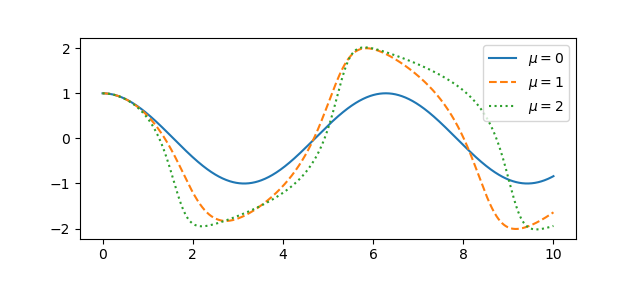A couple questions come to mind. First, how quickly do the solutions become periodic? Second, how does the period depend on μ? To address these questions, we’ll use an optional argument to ivp_solve we didn’t need in the earlier post.

## Using events in ivp_solve

For ivp_solve an event is a function of the time t and the solution y whose roots the solver will report. To determine the period, we’ll look at where the solution is zero; our event function is trivial since we want to find the roots of the solution itself.

Recall from the earlier post that we cast our second order ODE as a pair of first order ODEs, and so our solution is a vector, the function x and its derivative. So to find roots of the solution, we look at what the solver sees as the first component of the solver. So here’s our event function:

    def root(t, y): return y

Let’s set μ = 2 and find the zeros of the solution over the interval [0, 40], starting from the initial condition x(0) = 1, x‘(0) = 0.

    mu = 2
sol = solve_ivp(vdp, [0, 40], [1, 0], events=root)
zeros = sol.t_events


Here we reuse the vdp function from the previous post about the Van der Pol oscillator.

To estimate the period of the limit cycle we look at the spacing between zeros, and how that spacing is changing.

    spacing = zeros[1:] - zeros[:-1]
deltas = spacing[1:] - spacing[:-1]


If we plot the deltas we see that the zero spacings quickly approach a constant value. Zero crossings are half periods, so the period of the limit cycle is twice the limiting spacing between zeros.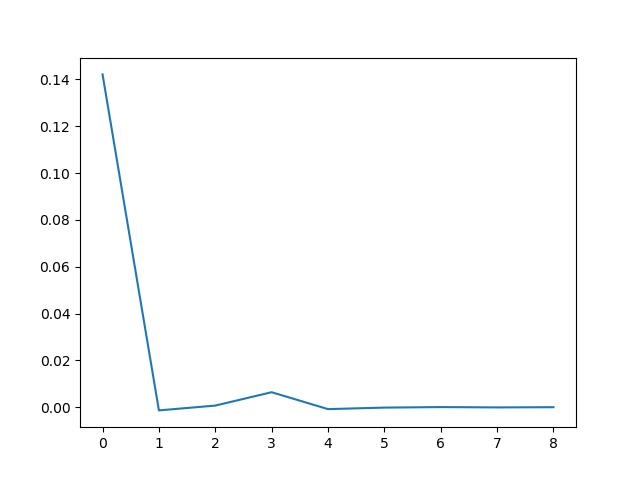## Theoretical results

If μ = 0 the Van der Pol oscillator reduces to a simple harmonic oscillator and the period is 2π. As μ increases, the period increases.

For relatively small μ we can calculate the period as above, but as μ increases this becomes more difficult numerically . But one can easily show that the period is asymptotically

T ~ (3 – 2 log 2) μ

as μ goes to infinity. A more refined estimate due to Mary Cartwright is

T ~ (3 – 2 log 2) μ + 2π/μ1/3

for large μ.

## More VdP posts

 There is a trivial solution, x = 0, corresponding to the initial conditions x(0) = x‘(0) = 0. Otherwise, every set of initial conditions leads to a solution that converges to the periodic attractor.

 To see why large values of μ are a problem numerically, here’s a plot of a solution for μ = 100.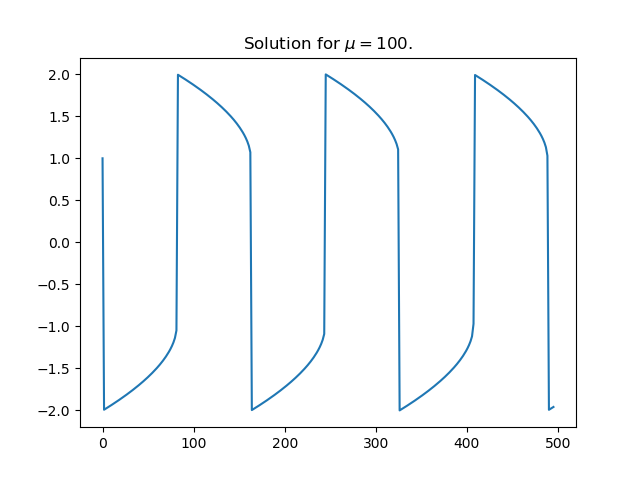The solution is differentiable everywhere, but the derivative changes so abruptly at the maxima and minima that it is discontinuous for practical purposes.

# Solving Van der Pol equation with ivp_solve

Van der Pol’s differential equation isThe equation describes a system with nonlinear damping, the degree of nonlinearity given by μ. If μ = 0 the system is linear and undamped, but as μ increases the strength of the nonlinearity increases. We will plot the phase portrait for the solution to Van der Pol’s equation in Python using SciPy’s new ODE solver ivp_solve.

The function ivp_solve does not solve second-order systems of equations directly. It solves systems of first-order equations, but a second-order differential equation can be recast as a pair of first-order equations by introducing the first derivative as a new variable.Since y is the derivative of x, the phase portrait is just the plot of (x, y).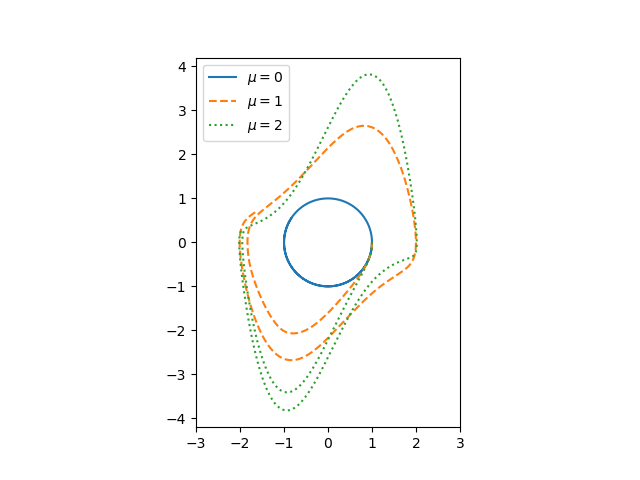If μ = 0, we have a simple harmonic oscillator and the phase portrait is simply a circle. For larger values of μ the solutions enter limiting cycles, but the cycles are more complicated than just circles. These limiting cycles are periodic attractors: every non-trivial solution converges to the limit cycle.

Here’s the Python code that made the plot.

from scipy import linspace
from scipy.integrate import solve_ivp
import matplotlib.pyplot as plt

def vdp(t, z):
x, y = z
return [y, mu*(1 - x**2)*y - x]

a, b = 0, 10

mus = [0, 1, 2]
styles = ["-", "--", ":"]
t = linspace(a, b, 500)

for mu, style in zip(mus, styles):
sol = solve_ivp(vdp, [a, b], [1, 0], t_eval=t)
plt.plot(sol.y, sol.y, style)

# make a little extra horizontal room for legend
plt.xlim([-3,3])
plt.legend([f"$\mu={m}$" for m in mus])
plt.axes().set_aspect(1)


To plot the solutions as a function of time, rather than plotting phase portraits, change the line

    plt.plot(sol.y, sol.y, style)

to

    plt.plot(sol.t, sol.y, style)

and comment out the line setting xlim This gives the following plot.# The other butterfly effect## The original butterfly effect

The butterfly effect is the semi-serious claim that a butterfly flapping its wings can cause a tornado half way around the world. It’s a poetic way of saying that some systems show sensitive dependence on initial conditions, that the slightest change now can make an enormous difference later. Often this comes up in the context of nonlinear, chaotic systems but it isn’t limited to that. I give an example here of a linear differential equation whose solutions start out the essentially the same but eventually diverge completely.

Once you think about these things for a while, you start to see nonlinearity and potential butterfly effects everywhere. There are tipping points everywhere waiting to be tipped!

## The other butterfly effect

But a butterfly flapping its wings usually has no effect, even in sensitive or chaotic systems. You might even say especially in sensitive or chaotic systems.

Sensitive systems are not always and everywhere sensitive to everything. They are sensitive in particular ways under particular circumstances, and can otherwise be quite resistant to influence. Not only may a system be insensitive to butterflies, it may even be relatively insensitive to raging bulls. The raging bull may have little more long-term effect than a butterfly. This is what I’m calling the other butterfly effect.

## Steering complex systems

In some ways chaotic systems are less sensitive to change than other systems. And this can be a good thing. Resistance to control also means resistance to unwanted tampering. Chaotic or stochastic systems can have a sort of self-healing property. They are more stable than more orderly systems, though in a different way.

The lesson that many people draw from their first exposure to complex systems is that there are high leverage points, if only you can find them and manipulate them. They want to insert a butterfly to at just the right time and place to bring about a desired outcome. Instead, we should humbly evaluate to what extent it is possible to steer complex systems at all. We should evaluate what aspects can be steered and how well they can be steered. The most effective intervention may not come from tweaking the inputs but from changing the structure of the system.